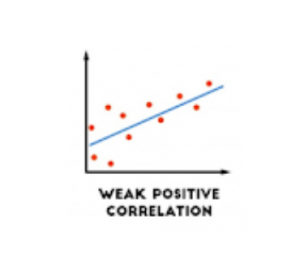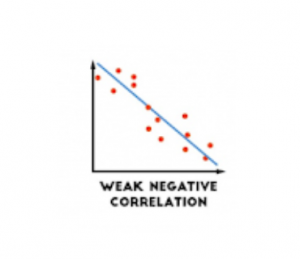# Interrelationships of quantitative variables: Correlation and Regression

Sometimes, in the experiment, we measure the two continuous characters which are associated with each other. For example, the height of plant and the temperature of the atmosphere. To understand the relationship between two such variables, we need to know how they are related and how the relationship can be expressed into a visual form.

### Correlation

The statistical technique to determine the relationship or association between two quantitatively measured or continuous variables is called correlation. It determines the relationship between two quantitative variables but it does not prove that one particular variable alone causes the change in the other.

### Correlation coefficient

The extent or degree of relationship between two sets of figures is measured in terms of another parameter called correlation coefficient. A simple correlation coefficient is denoted by the letter “r”. It is also known as Pearson’s correlation or product-moment correlation coefficient. For population coefficient, the Greek letter “ρ”, pronounced as “rho” is used. The absolute value of r remains constant irrespective of change of origin.

The extent of correlation varies between minus one and plus one. i.e. -1 ≤ r ≤ 1. It is expressed by decimal with + or – sign.

• The sign of “r” denotes the nature of the association.
• The Value of “r” denotes the strength of the association.

### Type of Correlation

Visual representation of bivariate data is simply given as a scatter diagram made on a graph paper by plotting each pair of variables (X and Y) by placing a dot at the point corresponding to the values of X and Y.

#### Perfect Positive Correlation

• The two variables are directly proportional and fully correlated with each other.
• The correlation coefficient (r) = +1.
• Both variables rise or fall at the same proportion.
• The graph forms a straight line rising from the lower ends of both X and Y axes.
• When the scatter diagram is drawn, all points fall on this straight line.

####• The two variables are inversely proportional to each other.
• The correlation coefficient (r) = -1.
• When one variable rises, the other variable falls at the same proportion.
• The graph will show a straight line starting from either of the extreme ends.
• When the scatter diagram is drawn, all points fall on this straight line.

#### Moderately or Partially Positive Correlation• The two variables are moderately proportional to one another.

• The correlation coefficient (r) = 0 < r < 1.
• The variables are moderately proportional. i.e. They rise and fall at similar proportion.
• The graph forms an imaginary line rising from the lower ends of both X and Y axes.
• When the scatter diagram is drawn, the points will scatter around an imaginary mean line.

#### Moderately or Partially Negative Correlation• The two variables are moderately inversely proportional to each other.
• The correlation coefficient (r) = -1< r< 0.
• The variables are moderately proportional. i.e. when one variable rises, the other variable falls at a similar proportion.
• The graph forms an imaginary line from either of the extreme ends.
• When the scatter diagram is drawn, the points will scatter around an imaginary mean line.

#### Absolutely No Correlation

• The two variables have no association with each other. i.e. no linear relationship.
• The correlation coefficient (r) = 0
• Both the variables rise or fall independently.
• The graph shows no imaginary line that indicates the trend of correlation.
• When the scattered diagram is drawn, the points will be much scattered.

#### Calculation of Correlation coefficient

This calculation is introduced by Professor Karl Pearson used to determine the direction and degree of linear relationship between two variables. The variables must be normally distributed for this method to be applied.

Formula:

Where numerator indicates covariability between two variables.

### Hypothesis testing from Pearson Correlation

As we carry out an experiment and take observations from sample, the observed value of “r” has to be tested for significance. The following formula is for the calculation of small sample. We set up null-hypothesis as:

Ho = There is no significant relationship between dependent and independent variables.

Formula:

where the degrees of freedom = n-2.

### Regression

We use regression analysis to describe the relationships between a set of independent variables and the dependent variable. Regression analysis produces a regression equation where the coefficients represent the relationship between each independent variable and the dependent variable. Thus it enables the user to predict the values of one variable on the basis of the other variable, on the positive or negative side, beyond the mean. The term “regression” was coined by Francis Galton in the nineteenth century to describe a biological phenomenon.

### Regression coefficient

Regression coefficient is denoted by the letter “b”. It shows the gradient or slope of the straight line of correlation. It is used to calculate the equation for a straight line in correlation (Y= a+bX).

Formula:

#### Plotting Graph

The calculated b is placed into the equation. Where “a” is a constant for Y-intercept, can also be found by subtracting the product of regression coefficient and mean of X from the mean of Y:

Then we plot a straight line placing the value of X and Y to find the points where the lines will go through.

Reference:

• Mahajan’s Methods in Biostatistics for Medical Students and Research Workers- page 219-269.
• Notes from Regression analysis and correlation by Prof. Rakha Hari Sarker.
• pictures from here